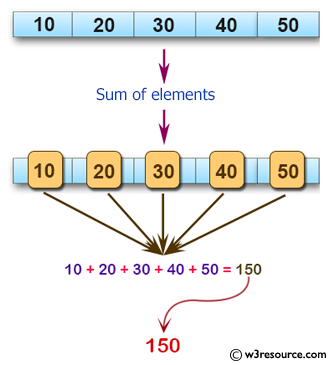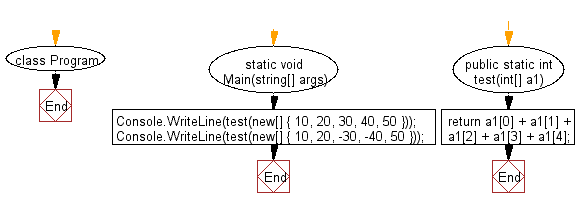﻿ C# - Compute the sum of the elements of an array of integers# C# Sharp Basic Algorithm Exercises: Compute the sum of the elements of an given array of integers

## C# Sharp Basic Algorithm: Exercise-91 with Solution

Write a C# Sharp program to compute the sum of the elements of an given array of integers.

Pictorial Presentation:Sample Solution:-

C# Sharp Code:

``````using System;
namespace exercises
{
class Program
{
static void Main(string[] args)
{
Console.WriteLine(test(new[] { 10, 20, 30, 40, 50 }));
Console.WriteLine(test(new[] { 10, 20, -30, -40, 50 }));
}
public static int  test(int[] a1)
{
return a1 + a1 + a1 + a1 + a1;
}
}
}
```
```

Sample Output:

```150
10```

Flowchart:C# Sharp Code Editor:

Improve this sample solution and post your code through Disqus

What is the difficulty level of this exercise?

Test your Programming skills with w3resource's quiz.

﻿